### Home > CALC > Chapter 3 > Lesson 3.1.1 > Problem3-16

3-16.
1.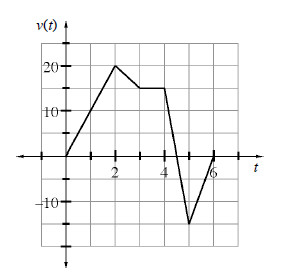After class, Stevie travels in a straight hallway with a velocity shown in the graph below right, where t is measured in minutes and v(t) is measured in feet per minute. Homework Help ✎

1. Explain what is happening when t > 4.5 minutes.

2. Calculate the total distance Stevie traveled.

3. If Stevie only travels in the straight hallway, how far does he end up from his original starting place?

4. What was Stevie's acceleration at t = 1?

5. When was Stevie's acceleration equal to zero?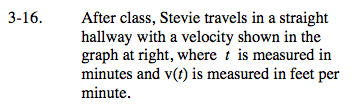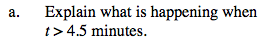Notice that the velocity is negative when t > 4.5.

When velocity is negative, Stevie moves back towards his starting position.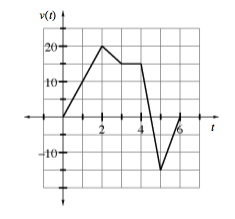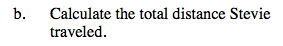Total distance is the area under a speed graph. Speed is the absolute value of velocity.

A speed graph is the absolute value of a velocity graph...
Area under a speed graph = total distance.
Area under a velocity graph = displacement.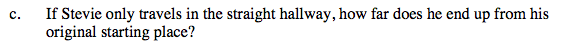Distance from starting point is known as 'displacement.'
Displacement is the area under a velocity graph.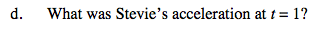Acceleration is slope on a velocity graph.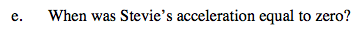See hint in part (d).